16 MAR 2021

# The impact of 0.1% daily profits on your investment.

It is commonly known that small steps lead to great results, but investors often tend to underestimate the importance of small percentages.
You might have heard that traders usually work for having big results in the shortest period of time, and this confuses many novice traders that invest with the unrealistic aim to become a millionaire in a few weeks starting with \$10 only.

In this article, I care to explain to you how you can achieve realistic returns by scoring just 0.1% profit per day.

You should see trading as a physical exercise. You cannot possibly try to lift a 100 kg bar on your first day at the gym, you might end up with some serious injuries and a wasted gym membership. Instead, you should start with a small bar and an ulterior load progressively in order to achieve realistic results.

The great thing about trading is that you can compound the return on your investment. How? Simply by reinvesting the full capital (initial + profits) every time you score profits.

How to calculate our profits after one investment in order to understand compounding?
I’ll try to keep it simple...

Let’s say that you invest 100€ in an interest-bearing bank that pays 5% interest annually, how much would you have at the end of the year?

The compounding formula is: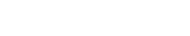FV=Future Value of the investment N periods from today
PV= The Present Value of our investment
r = The rate of interest per period

So with this formula, we can now obtain our Final Value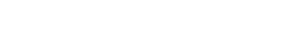Now that we know how to obtain a Final Value, what happens if we want to know how much it would be after 2 years?

Let’s assume that after every year we leave all the earnings in the bank, we can apply again the formula with the new Present Value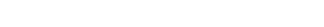But what if I want to know the Future Value after 5 years? We can still use the same formula but with a twist: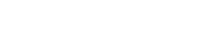N now represent the number of compounding periods.
So to know the Final Value after 5 years the formula is: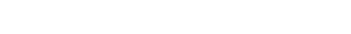You can use it also to calculate directly what we achieved previously with two formulas, just by adding the exponential at the end of the brackets.

How much 0.1% profit per day can impact your investment after a year?

Let’s use our formula with the new values, the interest rate of 0.1% and the compounding period of 365 days.

Note: The compounding period must always be correlated to the interest rate. If N is days, then r should be the one-day interest rate.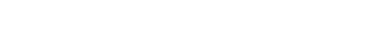With an investment of 100€ and a daily interest of 0.1%, you will end up with 144.03€ at the end of one year, congratulations!

Well actually no, you didn’t invest anything yet, did you?
But now you definitely know how small steps can lead to great results, in that case to 44.03% of profits after one year!

Could it work in the same way at the gym? You try and let me know.

It works for sure with Yanda Bots. Traders achieve on average 1.4% of profits with each Trading Bot started in less than 2 days.
How much would a 100€ investment worth after 1 year of trading with 1.4% every 2 days?
We do the math, you’ll make profits with Yanda.io :)

This tool is really handy for your Compound calculations
https://www.thecalculatorsite.com/finance/calculators/daily-compound-interest.php

Formulas and knowledge are taken from:
• DeFusco, Richard A. Quantitative Investment Analysis. Wiley, 2007.

Author: Francesco Greco